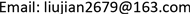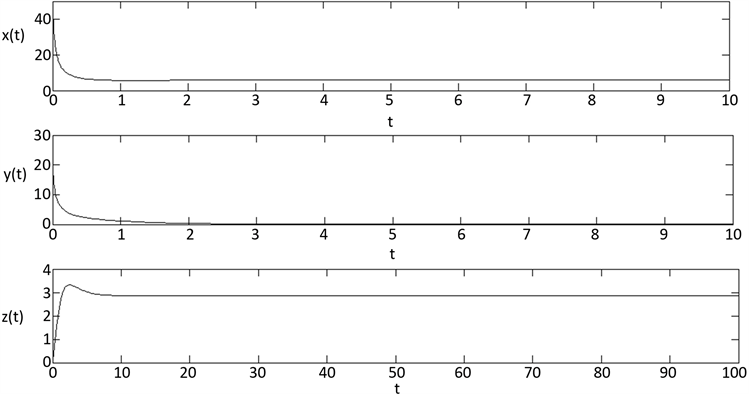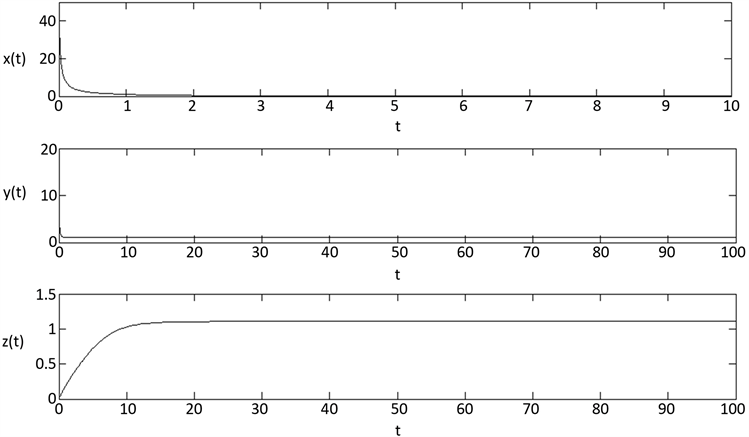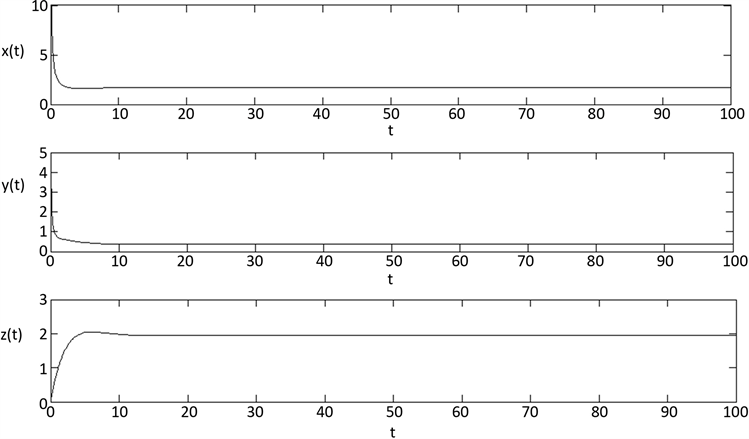﻿ 寨卡病毒溶瘤治疗脑癌的数学模型动力学分析 Dynamic Analysis of Mathematical Model in Brain Cancer Treatment by Zika Virus Oncolysis

Advances in Applied Mathematics
Vol. 08  No. 02 ( 2019 ), Article ID: 28970 , 15 pages
10.12677/AAM.2019.82032

Dynamic Analysis of Mathematical Model in Brain Cancer Treatment by Zika Virus Oncolysis

Tingmei Yang, Jian Liu

School of Mathematics and Information Science, Guangzhou University, Guangzhou GuangdongReceived: Jan. 30th, 2019; accepted: Feb. 14th, 2019; published: Feb. 22nd, 2019ABSTRACT

Based on the Zika Virus specifically targeting to kill glioma stem cells, and having no effect on normal cells, we build a mathematical model about normal cells and brain tumor cells competing nutrient in culture dish adding Zika Virus Oncolytic therapy by analyzing the existence and stability of the equilibrium, and get the minimum effective dose parameters expression when the Oncolytic virus therapy is the most effective. Finally, we can verify the result by numerical simulation.

Keywords:Zika Virus, Brain Cancer, Oncolytic Therapy, Stability, Drug Dose, Numerical SimulationCopyright © 2019 by authors and Hans Publishers Inc.

This work is licensed under the Creative Commons Attribution International License (CC BY).

http://creativecommons.org/licenses/by/4.0/1. 引言

2. 基础模型

2017年，王子子建立了一个基于细胞增长的抑制的溶瘤病毒治疗模型  。该文章在正常细胞和肿瘤细胞竞争的反应扩散模型基础上，结合算子半群理论推导，引入溶瘤病毒项，并得到肿瘤细胞根除平衡点的全局稳定性条件。具体模型如下：

$\left\{\begin{array}{l}{\partial }_{t}{u}_{1}\left(t,x\right)={d}_{1}\Delta {u}_{1}\left(t,x\right)+{u}_{1}\left(t,x\right)\left({a}_{1}-{b}_{1}{u}_{1}\left(t,x\right)-{c}_{1}{u}_{2}\left(t,x\right)\right),\text{\hspace{0.17em}}\text{\hspace{0.17em}}\text{\hspace{0.17em}}t>0,x\in \Omega \text{\hspace{0.17em}},\\ {\partial }_{t}{u}_{2}\left(t,x\right)={d}_{2}\Delta {u}_{2}\left(t,x\right)+{u}_{2}\left(t,x\right)\left({a}_{2}-{b}_{2}{u}_{1}\left(t,x\right)-{c}_{2}{u}_{2}\left(t,x\right)\right)\\ \text{\hspace{0.17em}}\text{\hspace{0.17em}}\text{\hspace{0.17em}}\text{\hspace{0.17em}}\text{\hspace{0.17em}}\text{\hspace{0.17em}}\text{\hspace{0.17em}}\text{\hspace{0.17em}}\text{\hspace{0.17em}}\text{\hspace{0.17em}}\text{\hspace{0.17em}}\text{\hspace{0.17em}}\text{\hspace{0.17em}}\text{\hspace{0.17em}}\text{\hspace{0.17em}}\text{\hspace{0.17em}}\text{\hspace{0.17em}}-\mu {u}_{2}\left(t,x\right){u}_{3}\left(t,x\right),\text{\hspace{0.17em}}\text{\hspace{0.17em}}\text{\hspace{0.17em}}t>0,x\in \Omega \text{\hspace{0.17em}},\\ {\partial }_{t}{u}_{3}\left(t,x\right)={d}_{3}\Delta {u}_{3}\left(t,x\right)+B-d{u}_{3}\left(t,x\right)+\gamma \mu {\int }_{\Omega }\Gamma \left(x,y,\tau \right){u}_{2}\left(t-\tau ,y\right){u}_{3}\left(t-\tau ,y\right)\text{d}y,\text{\hspace{0.17em}}t>0,x\in \Omega \text{\hspace{0.17em}},\\ {\partial }_{n}{u}_{1}={\partial }_{n}{u}_{2}={\partial }_{n}{u}_{3}=0,\text{\hspace{0.17em}}\text{\hspace{0.17em}}t>0,x\in \Omega \text{\hspace{0.17em}},\\ {u}_{1}\left(\theta ,x\right)={u}_{1}^{0}\left(\theta ,x\right),{u}_{2}\left(\theta ,x\right)={u}_{2}^{0}\left(\theta ,x\right),{u}_{3}\left(\theta ,x\right)={u}_{3}^{0}\left(\theta ,x\right),\text{\hspace{0.17em}}\text{\hspace{0.17em}}\theta \in \left[-\tau ,0\right],\text{\hspace{0.17em}}x\in \Omega \text{\hspace{0.17em}}.\end{array}$

$\left\{\begin{array}{l}\frac{dx}{dt}=x\left({a}_{1}-{b}_{1}x-{c}_{1}y\right),\\ \frac{dy}{dt}=y\left({a}_{2}-{b}_{2}y-{c}_{2}x\right)-{r}_{1}yz\text{\hspace{0.17em}},\\ \frac{dz}{dt}=B+{r}_{2}yz-dz\text{\hspace{0.17em}}.\end{array}$ (1)

3. 系统的非负性和有界性分析

$\left\{\begin{array}{l}\frac{dx}{dt}=x\left({a}_{1}-{b}_{1}x-{c}_{1}y\right),\\ \frac{dy}{dt}=y\left({a}_{2}-{b}_{2}y-{c}_{2}x\right).\end{array}$ (2)

$\left(0,0\right),\left(\frac{{a}_{1}}{{b}_{1}},0\right),\left(0,\frac{{a}_{2}}{{b}_{2}}\right),\left(\frac{{a}_{2}{c}_{1}-{a}_{1}{b}_{2}}{{c}_{1}{c}_{2}-{b}_{1}{b}_{2}},\frac{{a}_{1}{c}_{2}-{a}_{2}{b}_{1}}{{c}_{1}{c}_{2}-{b}_{1}{b}_{2}}\right).$

$l\equiv y+x-M,$

$M=\mathrm{max}\left\{\frac{{a}_{1}}{{b}_{1}},\frac{{a}_{2}}{{b}_{2}},\frac{{a}_{2}{c}_{1}-{a}_{1}{b}_{2}+{a}_{1}{c}_{2}-{a}_{2}{b}_{1}}{{c}_{1}{c}_{2}-{b}_{1}{b}_{2}}\right\}.$

$\begin{array}{l}\frac{\text{d}l}{\text{d}t}=\frac{\text{d}y}{\text{d}t}+\frac{\text{d}x}{\text{d}t}\\ \text{\hspace{0.17em}}\text{\hspace{0.17em}}\text{\hspace{0.17em}}\text{\hspace{0.17em}}=y\left({a}_{2}-{b}_{2}y-{c}_{2}x\right)+x\left({a}_{1}-{b}_{1}x-{c}_{1}y\right)\\ \text{\hspace{0.17em}}\text{\hspace{0.17em}}\text{\hspace{0.17em}}\text{\hspace{0.17em}}={\left(M-x\right)\left[{a}_{2}-{b}_{2}\left(M-x\right)-{c}_{2}x\right]+x\left[{a}_{1}-{b}_{1}x-{c}_{1}\left(M-x\right)\right]|}_{l=0}.\end{array}$

$\left\{\begin{array}{l}\frac{\text{d}y}{\text{d}t}=y\left({a}_{2}-{b}_{2}y-{c}_{2}x\right)-{r}_{1}yz\text{\hspace{0.17em}},\\ \frac{\text{d}z}{\text{d}t}=B+{r}_{2}yz-dz\text{\hspace{0.17em}}.\end{array}$

$L=y+\frac{{r}_{1}}{{r}_{2}}z,$

$\begin{array}{c}\frac{\text{d}L}{\text{d}t}=\frac{\text{d}y}{\text{d}t}+\frac{{r}_{1}}{{r}_{2}}\frac{\text{d}z}{\text{d}t}\\ =y\left({a}_{2}-{b}_{2}y-{c}_{2}x\right)-{r}_{1}yz+\frac{{r}_{1}}{{r}_{2}}\left(B+{r}_{2}yz-dz\right)\\ =y\left({a}_{2}-{b}_{2}y-{c}_{2}x\right)+\frac{{r}_{1}B}{{r}_{2}}-\frac{{r}_{1}}{{r}_{2}}dz\\ \le y\left({a}_{2}-mM\right)+\frac{{r}_{1}B}{{r}_{2}}-\frac{{r}_{1}}{{r}_{2}}dz\\ =\frac{{r}_{1}B}{{r}_{2}}-n\left(y+\frac{{r}_{1}}{{r}_{2}}z\right)=\frac{{r}_{1}B}{{r}_{2}}-nL\end{array}$

$\underset{t\to \infty }{\mathrm{lim}}\mathrm{sup}\left(y+\frac{{r}_{1}}{{r}_{2}}z\right)\le \frac{1}{n}\frac{{r}_{1}B}{{r}_{2}}.$

$m=\mathrm{min}\left\{{b}_{2},{c}_{2}\right\},n=\mathrm{min}\left\{|{a}_{2}-nm|,d\right\}.$

$\Gamma =\left\{\left(x,y,z\right)|x+y\le M,y+\frac{{r}_{1}}{{r}_{2}}z\le \frac{1}{n}\frac{{r}_{1}B}{{r}_{2}}\right\}.$

4. 平衡点的存在性与稳定性分析

2) 当 $B>\frac{d}{{r}_{1}}\left({a}_{2}-\frac{{a}_{1}{c}_{2}}{{b}_{1}}\right)$ 时，系统(1)除了存在平凡平衡点 ${E}_{0}\left(0,0,\frac{d}{B}\right)$，还存在肿瘤灭绝平衡点 ${E}_{1}\left(\frac{{a}_{1}}{{b}_{1}},0,\frac{B}{d}\right)$

3) 当 $B<\frac{d{a}_{2}}{{r}_{1}}$$\frac{{a}_{1}}{{c}_{1}}<{y}_{2}<\mathrm{min}\left\{\frac{{a}_{2}}{{b}_{2}},\frac{d}{{r}_{2}}\right\}$ 时，除了存在平凡平衡点 ${E}_{0}\left(0,0,\frac{d}{B}\right)$，肿瘤灭绝平衡点 ${E}_{1}\left(\frac{{a}_{1}}{{b}_{1}},0,\frac{B}{d}\right)$，还存在正常细胞灭绝平衡点 ${E}_{2}\left(0,{y}_{2},\frac{{a}_{2}-{b}_{2}{y}_{2}}{{r}_{1}}\right)$，其中

${y}_{2}=\frac{{a}_{2}{r}_{2}+{b}_{2}d-\sqrt{{\left({a}_{2}{r}_{2}+{b}_{2}d\right)}^{2}-4{r}_{2}{b}_{2}\left({a}_{2}d-B{r}_{1}\right)}}{2{b}_{2}{r}_{2}}$

4) 当 $B<\frac{d}{{r}_{1}}\left({a}_{2}-\frac{{a}_{1}{c}_{2}}{{b}_{1}}\right)$${c}_{1}{c}_{2}<{b}_{1}{b}_{2},{a}_{1}{c}_{2}<{a}_{2}{b}_{1},{y}_{3}<\mathrm{min}\left\{\frac{{a}_{1}}{{c}_{1}},\frac{d}{{r}_{2}}\right\}$ 时，除了存在平凡平衡点 ${E}_{0}\left(0,0,\frac{d}{B}\right)$ 和肿瘤灭绝平衡点 ${E}_{1}\left(\frac{{a}_{1}}{{b}_{1}},0,\frac{B}{d}\right)$，还存在正平衡点 ${E}_{3}\left(\frac{{a}_{1}-{c}_{1}{y}_{3}}{{b}_{1}},{y}_{3},\frac{B}{d-{r}_{2}{y}_{3}}\right)$，其中

${y}_{3}=\frac{-{h}_{2}-\sqrt{{h}_{2}^{2}-4{h}_{1}{h}_{3}}}{2{h}_{1}},$

${h}_{1}={b}_{1}{b}_{2}{r}_{2}-{c}_{1}{c}_{2}{r}_{2},{h}_{2}={c}_{1}{c}_{2}d+{a}_{1}{c}_{2}{r}_{2}-{a}_{2}{b}_{1}{r}_{2}-{b}_{1}{b}_{2}d,{h}_{3}={a}_{2}{b}_{1}d-{r}_{1}{b}_{1}B-{a}_{1}{c}_{2}d.$

3) 当 $y>\frac{{a}_{1}}{{c}_{1}}$ 时，溶瘤病毒治疗最终失败，有 $x=0$ 得到 $\left\{\begin{array}{l}{a}_{2}-{b}_{2}y-{r}_{1}z=0\\ B+{r}_{2}yz-dz=0\end{array}$。要使平衡点 ${z}_{2}$ 存在，由该方程组的第一个式子知， ${a}_{2}-{b}_{2}{y}_{2}>0$ 则必有 ${y}_{2}<\frac{{a}_{2}}{{b}_{2}}$，由方程组的第二个式子知， ${r}_{2}{y}_{2}-d<0$ 则必有 ${y}_{2}<\frac{d}{{r}_{2}}$，故 ${y}_{2}<\mathrm{min}\left\{\frac{{a}_{2}}{{b}_{2}},\frac{d}{{r}_{2}}\right\}$。把 $z=\frac{{a}_{2}-{b}_{2}y}{{r}_{1}}$ 代入方程组得到

${r}_{2}{b}_{2}{y}^{2}-\left({a}_{2}{r}_{2}+{b}_{2}d\right)y+{a}_{2}d-B{r}_{1}=0.$

$f\left(y\right)={r}_{2}{b}_{2}{y}^{2}-\left({a}_{2}{r}_{2}+{b}_{2}d\right)y+{a}_{2}d-B{r}_{1},$

$B<\frac{{a}_{2}d}{{r}_{1}}$，则 ${y}_{1}^{\ast }{y}_{2}^{\ast }=\frac{{a}_{2}d-B{r}_{1}}{{r}_{2}{b}_{2}}$，即进一步假设 $\frac{{a}_{2}}{{b}_{2}}\le \frac{d}{{r}_{2}}$，则函数 $f\left(y\right)$ 的对称轴 $y=\frac{1}{2}\left(\frac{{a}_{2}}{{b}_{2}}+\frac{d}{{r}_{2}}\right)\le \frac{{a}_{2}}{{b}_{2}}$

$f\left(0\right)={a}_{2}d-B{r}_{1}>0,$

$f\left(\frac{{a}_{2}}{{b}_{2}}\right)={r}_{2}{b}_{2}{\left(\frac{{a}_{2}}{{b}_{2}}\right)}^{2}-\left({a}_{2}{r}_{2}+{b}_{2}d\right)\frac{{a}_{2}}{{b}_{2}}+{a}_{2}d-B{r}_{1}=\frac{{r}_{2}{a}_{2}^{2}}{{b}_{2}}-\frac{{a}_{2}^{2}{r}_{2}}{{b}_{2}}-{a}_{2}d+{a}_{2}d-B{r}_{1}=-B{r}_{1}<0.$

$f\left(y\right)$$\left(0,\frac{{a}_{2}}{{b}_{2}}\right)$ 有一实数根。

$f\left(\frac{d}{{r}_{2}}\right)={r}_{2}{b}_{2}{\left(\frac{d}{{r}_{2}}\right)}^{2}-\left({a}_{2}{r}_{2}+{b}_{2}d\right)\frac{d}{{r}_{2}}+{a}_{2}d-B{r}_{1}=\frac{{b}_{2}{d}^{2}}{{r}_{2}}-\frac{{d}^{2}{b}_{2}}{{r}_{2}}-{a}_{2}d+{a}_{2}d-B{r}_{1}=-B{r}_{1}<0.$

$f\left(y\right)$$\left(0,\frac{d}{{r}_{2}}\right)$ 有一实数根。若 $B\ge \frac{{a}_{2}d}{{r}_{1}}$，此时有 $f\left(0\right)={a}_{2}d-B{r}_{1}<0$，且 $f\left(\frac{{a}_{2}}{{b}_{2}}\right)<0,f\left(\frac{d}{{r}_{2}}\right)<0$，故 $f\left(y\right)$$\left(0,\frac{{a}_{2}}{{b}_{2}}\right)$$\left(0,\frac{d}{{r}_{2}}\right)$ 均无解。由以上讨论可知，当满足 $B<\frac{d}{{r}_{1}}{a}_{2}$$\frac{{a}_{1}}{{c}_{1}}<{y}_{2}<\mathrm{min}\left\{\frac{{a}_{2}}{{b}_{2}},\frac{d}{{r}_{2}}\right\}$ 时，系统(1)存在正常细胞灭绝平衡点 ${E}_{2}\left(0,{y}_{2},\frac{{a}_{2}-{b}_{2}{y}_{2}}{{r}_{1}}\right)$，其中

${y}_{2}=\frac{{a}_{2}{r}_{2}+{b}_{2}d-\sqrt{{\left({a}_{2}{r}_{2}+{b}_{2}d\right)}^{2}-4{r}_{2}{b}_{2}\left({a}_{2}d-B{r}_{1}\right)}}{2{b}_{2}{r}_{2}}.$

4) 由 $\left\{\begin{array}{l}{a}_{1}-{b}_{1}x-{c}_{1}y=0\\ {a}_{2}-{b}_{2}y-{c}_{2}x-{r}_{1}z=0\\ B+{r}_{2}yz-dz=0\end{array}$ 可知 $\left\{\begin{array}{l}x=\frac{{a}_{1}-{c}_{1}y}{{b}_{1}}\\ z=\frac{B}{d-{r}_{2}y}\end{array}$，要使平衡点 ${x}_{3},{z}_{3}$ 存在，则必有 ${a}_{1}-{c}_{1}{y}_{3}>0$$d-{r}_{2}{y}_{3}>0$。即 $\left\{\begin{array}{l}{y}_{3}<\frac{{a}_{1}}{{c}_{1}}\\ {y}_{3}<\frac{d}{{r}_{2}}\end{array}$，故 ${y}_{3}<\mathrm{min}\left\{\frac{{a}_{1}}{{c}_{1}},\frac{d}{{r}_{2}}\right\}$。接下来讨论 ${y}_{3}$ 平衡点 ${y}_{3}$ 满足方程

$\left({b}_{1}{b}_{2}{r}_{2}-{c}_{1}{c}_{2}{r}_{2}\right){y}_{3}^{2}+\left({c}_{1}{c}_{2}d+{a}_{1}{c}_{2}{r}_{2}-{a}_{2}{b}_{1}{r}_{2}-{b}_{1}{b}_{2}d\right){y}_{3}+{a}_{2}{b}_{1}d-{r}_{1}{b}_{1}B-{a}_{1}{c}_{2}d=0$

$\begin{array}{l}{h}_{1}={b}_{1}{b}_{2}{r}_{2}-{c}_{1}{c}_{2}{r}_{2},{h}_{2}={c}_{1}{c}_{2}d+{a}_{1}{c}_{2}{r}_{2}-{a}_{2}{b}_{1}{r}_{2}-{b}_{1}{b}_{2}d,\\ {h}_{3}={a}_{2}{b}_{1}d-{r}_{1}{b}_{1}B-{a}_{1}{c}_{2}d.\end{array}$

$f\left(y\right)=\left({b}_{1}{b}_{2}{r}_{2}-{c}_{1}{c}_{2}{r}_{2}\right){y}^{2}+\left({c}_{1}{c}_{2}d+{a}_{1}{c}_{2}{r}_{2}-{a}_{2}{b}_{1}{r}_{2}-{b}_{1}{b}_{2}d\right)y+\left({a}_{2}{b}_{1}d-{r}_{1}{b}_{1}B-{a}_{1}{c}_{2}d\right),$

① 当 ${c}_{1}{c}_{2}<{b}_{1}{b}_{2},{a}_{1}{c}_{2}<{a}_{2}{b}_{1}$$0 时，则 ${h}_{1}>0,{h}_{2}<0,{h}_{3}>0$，进一步假设 $\frac{{a}_{1}}{{c}_{1}}\le \frac{d}{{r}_{2}}$，则函数 $f\left(y\right)$ 的对称轴

$y=\frac{1}{2}\left(\frac{{c}_{1}{c}_{2}d+{a}_{1}{c}_{2}{r}_{2}-{a}_{2}{b}_{1}{r}_{2}-{b}_{1}{b}_{2}d}{\left({c}_{1}{c}_{2}-{b}_{1}{b}_{2}\right){r}_{2}}\right)=\frac{1}{2}\left(\frac{d}{{r}_{2}}+\frac{{a}_{1}{c}_{2}-{a}_{2}{b}_{1}}{{c}_{1}{c}_{2}-{b}_{1}{b}_{2}}\right)\le \frac{{a}_{1}}{{c}_{1}}.$

$\begin{array}{c}f\left(\frac{{a}_{1}}{{c}_{1}}\right)=\left({b}_{1}{b}_{2}{r}_{2}-{c}_{1}{c}_{2}{r}_{2}\right){\left(\frac{{a}_{1}}{{c}_{1}}\right)}^{2}+\left({c}_{1}{c}_{2}d+{a}_{1}{c}_{2}{r}_{2}-{a}_{2}{b}_{1}{r}_{2}-{b}_{1}{b}_{2}d\right)\frac{{a}_{1}}{{c}_{1}}\\ \text{\hspace{0.17em}}\text{\hspace{0.17em}}+\left({a}_{2}{b}_{1}d-{r}_{1}{b}_{1}B-{a}_{1}{c}_{2}d\right)\\ ={b}_{1}d\left({a}_{2}-\frac{{a}_{1}{b}_{2}}{{c}_{1}}\right)+{b}_{1}{r}_{2}\frac{{a}_{1}}{{c}_{1}}\left(\frac{{a}_{1}{b}_{2}}{{c}_{1}}-{a}_{2}\right)-{r}_{1}{b}_{1}B\\ ={b}_{1}\left(\frac{{a}_{1}{b}_{2}}{{c}_{1}}-{a}_{2}\right)\left({r}_{2}\frac{{a}_{1}}{{c}_{1}}-d\right)-{r}_{1}{b}_{1}B.\end{array}$

$\frac{{a}_{1}}{{c}_{1}}\le \frac{d}{{r}_{2}}$，得 $\frac{{r}_{2}{a}_{1}}{{c}_{1}}-d<0$，由 $\frac{1}{2}\left(\frac{d}{{r}_{2}}+\frac{{a}_{1}{c}_{2}-{a}_{2}{b}_{1}}{{c}_{1}{c}_{2}-{b}_{1}{b}_{2}}\right)\le \frac{{a}_{1}}{{c}_{1}}$$\frac{{a}_{1}}{{c}_{1}}\le \frac{d}{{r}_{2}}$，得 $\frac{{a}_{1}{b}_{2}}{{c}_{1}}-{a}_{2}>0$。所以有 $f\left(\frac{{a}_{1}}{{c}_{1}}\right)<0$

$0，因此 $f\left(0\right)={a}_{2}{b}_{1}d-{r}_{1}{b}_{1}B-{a}_{1}{c}_{2}d>0$。故 $f\left(y\right)$$\left(0,\frac{{a}_{1}}{{c}_{1}}\right)$ 有实数根。

$y=\frac{1}{2}\frac{{c}_{1}{c}_{2}d+{a}_{1}{c}_{2}{r}_{2}-{a}_{2}{b}_{1}{r}_{2}-{b}_{1}{b}_{2}d}{\left({c}_{1}{c}_{2}-{b}_{1}{b}_{2}\right){r}_{2}}=\frac{1}{2}\left(\frac{d}{{r}_{2}}+\frac{{a}_{1}{c}_{2}-{a}_{2}{b}_{1}}{{c}_{1}{c}_{2}-{b}_{1}{b}_{2}}\right)\le \frac{d}{{r}_{2}},$

$\begin{array}{c}f\left(\frac{d}{{r}_{2}}\right)=\left({b}_{1}{b}_{2}{r}_{2}-{c}_{1}{c}_{2}{r}_{2}\right){\left(\frac{d}{{r}_{2}}\right)}^{2}+\left({c}_{1}{c}_{2}d+{a}_{1}{c}_{2}{r}_{2}-{a}_{2}{b}_{1}{r}_{2}-{b}_{1}{b}_{2}d\right)\frac{d}{{r}_{2}}\\ \text{\hspace{0.17em}}\text{\hspace{0.17em}}+\left({a}_{2}{b}_{1}d-{r}_{1}{b}_{1}B-{a}_{1}{c}_{2}d\right)\\ =-{r}_{1}{b}_{1}B<0.\end{array}$

$0，因此 $f\left(0\right)={a}_{2}{b}_{1}d-{r}_{1}{b}_{1}B-{a}_{1}{c}_{2}d>0$。故 $f\left(y\right)$$\left(0,\frac{d}{{r}_{2}}\right)$ 有实数根。

② 当 ${c}_{1}{c}_{2}>{b}_{1}{b}_{2}$$B>\frac{d}{{r}_{1}}\left({a}_{2}-\frac{{a}_{1}{c}_{2}}{{b}_{1}}\right)$ 时， ${h}_{1}<0,{h}_{3}<0$，则

$f\left(0\right)={a}_{2}{b}_{1}d-{r}_{1}{b}_{1}B-{a}_{1}{c}_{2}d<0.$

$\frac{{a}_{1}}{{c}_{1}}\le \frac{d}{{r}_{2}}$，则函数 $f\left(y\right)$ 的对称轴

$y=\frac{1}{2}\frac{{c}_{1}{c}_{2}d+{a}_{1}{c}_{2}{r}_{2}-{a}_{2}{b}_{1}{r}_{2}-{b}_{1}{b}_{2}d}{\left({c}_{1}{c}_{2}-{b}_{1}{b}_{2}\right){r}_{2}}=\frac{1}{2}\left(\frac{d}{{r}_{2}}+\frac{{a}_{1}{c}_{2}-{a}_{2}{b}_{1}}{{c}_{1}{c}_{2}-{b}_{1}{b}_{2}}\right)\le \frac{{a}_{1}}{{c}_{1}},$

$\begin{array}{c}f\left(\frac{{a}_{1}}{{c}_{1}}\right)=\left({b}_{1}{b}_{2}{r}_{2}-{c}_{1}{c}_{2}{r}_{2}\right){\left(\frac{{a}_{1}}{{c}_{1}}\right)}^{2}+\left({c}_{1}{c}_{2}d+{a}_{1}{c}_{2}{r}_{2}-{a}_{2}{b}_{1}{r}_{2}-{b}_{1}{b}_{2}d\right)\frac{{a}_{1}}{{c}_{1}}+\left({a}_{2}{b}_{1}d-{r}_{1}{b}_{1}B-{a}_{1}{c}_{2}d\right)\\ ={b}_{1}d\left({a}_{2}-\frac{{a}_{1}{b}_{2}}{{c}_{1}}\right)+{b}_{1}{r}_{2}\frac{{a}_{1}}{{c}_{1}}\left(\frac{{a}_{1}{b}_{2}}{{c}_{1}}-{a}_{2}\right)-{r}_{1}{b}_{1}B\\ ={b}_{1}\left(\frac{{a}_{1}{b}_{2}}{{c}_{1}}-{a}_{2}\right)\left({r}_{2}\frac{{a}_{1}}{{c}_{1}}-d\right)-{r}_{1}{b}_{1}B<0.\end{array}$

$f\left(y\right)$$\left(0,\frac{{a}_{1}}{{c}_{1}}\right)$ 无实根。

$\frac{{a}_{1}}{{c}_{1}}\ge \frac{d}{{r}_{2}}$，则函数 $f\left(y\right)$ 的对称轴

$y=\frac{1}{2}\frac{{c}_{1}{c}_{2}d+{a}_{1}{c}_{2}{r}_{2}-{a}_{2}{b}_{1}{r}_{2}-{b}_{1}{b}_{2}d}{\left({c}_{1}{c}_{2}-{b}_{1}{b}_{2}\right){r}_{2}}=\frac{1}{2}\left(\frac{d}{{r}_{2}}+\frac{{a}_{1}{c}_{2}-{a}_{2}{b}_{1}}{{c}_{1}{c}_{2}-{b}_{1}{b}_{2}}\right)\le \frac{d}{{r}_{2}},$

$\begin{array}{c}f\left(\frac{d}{{r}_{2}}\right)=\left({b}_{1}{b}_{2}{r}_{2}-{c}_{1}{c}_{2}{r}_{2}\right){\left(\frac{d}{{r}_{2}}\right)}^{2}+\left({c}_{1}{c}_{2}d+{a}_{1}{c}_{2}{r}_{2}-{a}_{2}{b}_{1}{r}_{2}-{b}_{1}{b}_{2}d\right)\frac{d}{{r}_{2}}+\left({a}_{2}{b}_{1}d-{r}_{1}{b}_{1}B-{a}_{1}{c}_{2}d\right)\\ =-{r}_{1}{b}_{1}B<0.\end{array}$

$f\left(y\right)$$\left(0,\frac{d}{{r}_{2}}\right)$ 无实根。因此，当 $B<\frac{d}{{r}_{1}}\left({a}_{2}-\frac{{a}_{1}{c}_{2}}{{b}_{1}}\right)$${c}_{1}{c}_{2}<{b}_{1}{b}_{2},{a}_{1}{c}_{2}<{a}_{2}{b}_{1},{y}_{3}<\mathrm{min}\left\{\frac{{a}_{1}}{{c}_{1}},\frac{d}{{r}_{2}}\right\}$ 时，系统(1)存在一正平衡点 ${E}_{3}\left(\frac{{a}_{1}-{c}_{1}{y}_{3}}{{b}_{1}},{y}_{3},\frac{B}{d-{r}_{2}{y}_{3}}\right)$，其中 ${y}_{3}=\frac{-{h}_{2}-\sqrt{{h}_{2}^{2}-4{h}_{1}{h}_{3}}}{2{h}_{1}}$

$J\left(E\right)=\left(\begin{array}{ccc}{a}_{1}-2{b}_{1}x-{c}_{1}y& -{c}_{1}x& 0\\ -{c}_{2}y& {a}_{2}-2{b}_{2}y-{c}_{2}x-{r}_{1}z& -{r}_{1}y\\ 0& {r}_{2}z& {r}_{2}y-d\end{array}\right).$

$J\left({E}_{0}\right)=\left(\begin{array}{ccc}{a}_{1}& 0& 0\\ 0& {a}_{2}-\frac{{r}_{1}B}{d}& 0\\ 0& \frac{{r}_{2}B}{d}& -d\end{array}\right).$

$\left(\lambda -{a}_{1}\right)\left(\lambda -{a}_{2}+\frac{{r}_{1}B}{d}\right)\left(\lambda +d\right)=0.$

$J\left({E}_{1}\right)=\left(\begin{array}{ccc}-{a}_{1}& -\frac{{a}_{1}{c}_{1}}{{b}_{1}}& 0\\ 0& {a}_{2}-\frac{{a}_{1}{c}_{2}}{{b}_{1}}-\frac{{r}_{1}B}{d}& 0\\ 0& \frac{{r}_{2}B}{d}& -d\end{array}\right)\text{\hspace{0.17em}}.$

$\left(\lambda +{a}_{1}\right)\left(\lambda -{a}_{2}+\frac{{a}_{1}{c}_{2}}{{b}_{1}}+\frac{{r}_{1}B}{d}\right)\left(\lambda +d\right)=0.$

${\lambda }_{1}=-{a}_{1},{\lambda }_{2}={a}_{2}-\frac{{a}_{1}{c}_{2}}{{b}_{1}}-\frac{{r}_{1}B}{d},{\lambda }_{3}=-d.$

$B>\frac{d}{{r}_{1}}\left({a}_{2}-\frac{{a}_{1}{c}_{2}}{{b}_{1}}\right)$ 时，特征根 ${\lambda }_{2}<0$，又由于 ${\lambda }_{1}<0,{\lambda }_{3}<0$，故肿瘤灭绝平衡点 ${E}_{1}\left(\frac{{a}_{1}}{{b}_{1}},0,\frac{B}{d}\right)$ 是局部渐近稳定的。此外，当 $B<\frac{d}{{r}_{1}}\left({a}_{2}-\frac{{a}_{1}{c}_{2}}{{b}_{1}}\right)$ 时，显然有特征根 ${\lambda }_{2}>0$，从而肿瘤灭绝平衡 ${E}_{1}\left(\frac{{a}_{1}}{{b}_{1}},0,\frac{B}{d}\right)$ 是不稳定的。

$\left\{\begin{array}{l}\frac{\text{d}x}{\text{d}t}=x\left({a}_{1}-{b}_{1}x\right),\\ \frac{\text{d}z}{\text{d}t}=B-dz\text{\hspace{0.17em}}.\end{array}$ (3)

$\frac{\frac{1}{xz}\left({a}_{1}x-{b}_{1}{x}^{2}\right)}{\partial x}+\frac{\frac{1}{xz}\left(B-dz\right)}{\partial z}=-\frac{{b}_{1}}{z}-\frac{B}{x{z}^{2}}<0.$

$J\left({E}_{2}\right)=\left(\begin{array}{ccc}{a}_{1}-{c}_{1}{y}_{2}& 0& 0\\ -{c}_{2}{y}_{2}& -{b}_{2}{y}_{2}& -{r}_{1}{y}_{2}\\ 0& {r}_{2}\frac{{a}_{2}-{b}_{2}{y}_{2}}{{r}_{1}}& {r}_{2}{y}_{2}-d\end{array}\right).$

$\left(\lambda -{a}_{1}+{c}_{1}{y}_{2}\right)\left[\left(\lambda +{b}_{2}{y}_{2}\right)\left(\lambda -{r}_{2}{y}_{2}+d\right)+{r}_{2}{y}_{2}\left({a}_{2}-{b}_{2}{y}_{2}\right)\right]=0.$

${\lambda }^{2}+\left({b}_{2}{y}_{2}+d-{r}_{2}{y}_{2}\right)\lambda +\left({b}_{2}d{y}_{2}+{a}_{2}{r}_{2}{y}_{2}-2{b}_{2}{r}_{2}{y}_{2}^{2}\right)=0.$

${y}_{2}<\frac{d}{{r}_{2}}$${y}_{2}<\frac{{a}_{2}}{{b}_{2}}$ 得到

${\lambda }_{2}+{\lambda }_{3}={r}_{2}{y}_{2}-{b}_{2}{y}_{2}-d<0,$

${\lambda }_{2}{\lambda }_{3}={b}_{2}d{y}_{2}+{a}_{2}{r}_{2}{y}_{2}-2{b}_{2}{r}_{2}{y}_{2}^{2}={y}_{2}\left[{b}_{2}\left(d-{r}_{2}{y}_{2}\right)+{r}_{2}\left({a}_{2}-{b}_{2}{y}_{2}\right)\right]>0.$

${\lambda }_{2}<0,{\lambda }_{3}<0$。因此，当 $B<\frac{d}{{r}_{1}}{a}_{2}$$\frac{{a}_{1}}{{c}_{1}}<{y}_{2}<\mathrm{min}\left\{\frac{{a}_{2}}{{b}_{2}},\frac{d}{{r}_{2}}\right\}$ 时正常细胞灭绝平衡点 ${E}_{2}\left(0,{y}_{2},\frac{{a}_{2}-{b}_{2}{y}_{2}}{{r}_{1}}\right)$ 是局部渐近稳定的。

$\left\{\begin{array}{l}\frac{\text{d}y}{\text{d}t}=y\left({a}_{2}-{b}_{2}y-{r}_{1}z\right),\\ \frac{\text{d}z}{\text{d}t}=B+{r}_{2}yz-dz\text{\hspace{0.17em}}\text{​}.\end{array}$ (4)

$\frac{\frac{1}{yz}y\left({a}_{2}-{b}_{2}y-{r}_{1}z\right)}{\partial y}+\frac{\frac{1}{yz}\left(B+{r}_{2}yz-dz\right)}{\partial z}=-\frac{{b}_{2}}{z}-\frac{B}{y{z}^{2}}<0.$

$J\left({E}_{3}\right)=\left(\begin{array}{ccc}{c}_{1}{y}_{3}-{a}_{1}& -\frac{{c}_{1}\left({a}_{1}-{c}_{1}{y}_{3}\right)}{{b}_{1}}& 0\\ -{c}_{2}{y}_{3}& {a}_{2}-2{b}_{2}{y}_{3}-\frac{{c}_{2}\left({a}_{1}-{c}_{1}{y}_{3}\right)}{{b}_{1}}-\frac{B{r}_{1}}{d-{r}_{2}{y}_{3}}& -{r}_{1}{y}_{3}\\ 0& \frac{B{r}_{2}}{d-{r}_{2}{y}_{3}}& {r}_{2}{y}_{3}-d\end{array}\right).$

${\lambda }^{3}+{e}_{1}{\lambda }^{2}+{e}_{2}\lambda +{e}_{3}=0.$

$\begin{array}{l}{e}_{1}=-\left[\left({r}_{2}{y}_{3}-d\right)+\left({a}_{2}-2{b}_{2}{y}_{3}-\frac{{c}_{2}\left({a}_{1}-{c}_{1}{y}_{3}\right)}{{b}_{1}}-\frac{B{r}_{1}}{d-{r}_{2}{y}_{3}}\right)+\left({c}_{1}{y}_{3}-{a}_{1}\right)\right]\\ \text{\hspace{0.17em}}\text{\hspace{0.17em}}\text{\hspace{0.17em}}=\left({a}_{1}-{c}_{1}{y}_{3}\right)+\left(d-{r}_{2}{y}_{3}\right)+{y}_{3}\left({b}_{2}-\frac{{c}_{1}{c}_{2}}{{b}_{1}}\right)\\ \text{\hspace{0.17em}}\text{\hspace{0.17em}}\text{\hspace{0.17em}}\text{\hspace{0.17em}}\text{\hspace{0.17em}}+\left[\frac{{a}_{1}{c}_{2}}{{b}_{1}}-\left({a}_{2}-{b}_{2}{y}_{3}-\frac{B{r}_{1}}{d-{r}_{2}{y}_{3}}\right)\right]>0.\end{array}$

$\begin{array}{l}{e}_{2}=\left({a}_{2}-2{b}_{2}{y}_{3}-\frac{{c}_{2}\left({a}_{1}-{c}_{1}{y}_{3}\right)}{{b}_{1}}-\frac{B{r}_{1}}{d-{r}_{2}{y}_{3}}\right)\left({r}_{2}{y}_{3}-d\right)+\frac{B{r}_{2}}{d-{r}_{2}{y}_{3}}{r}_{1}{y}_{3}+\left({c}_{1}{y}_{3}-{a}_{1}\right)\left({r}_{2}{y}_{3}-d\right)\\ \text{\hspace{0.17em}}\text{\hspace{0.17em}}\text{\hspace{0.17em}}\text{\hspace{0.17em}}\text{\hspace{0.17em}}\text{\hspace{0.17em}}\text{ }+\left({a}_{2}-2{b}_{2}{y}_{3}-\frac{{c}_{2}\left({a}_{1}-{c}_{1}{y}_{3}\right)}{{b}_{1}}-\frac{B{r}_{1}}{d-{r}_{2}{y}_{3}}\right)\left({c}_{1}{y}_{3}-{a}_{1}\right)+{c}_{2}{y}_{3}\frac{{c}_{1}\left({a}_{1}-{c}_{1}{y}_{3}\right)}{{b}_{1}}\\ \text{\hspace{0.17em}}\text{\hspace{0.17em}}\text{\hspace{0.17em}}\text{\hspace{0.17em}}\text{\hspace{0.17em}}\text{\hspace{0.17em}}\text{ }=\left({a}_{2}-2{b}_{2}{y}_{3}-\frac{{c}_{2}\left({a}_{1}-{c}_{1}{y}_{3}\right)}{{b}_{1}}-\frac{B{r}_{1}}{d-{r}_{2}{y}_{3}}\right)\left({r}_{2}{y}_{3}-d+{c}_{1}{y}_{3}-{a}_{1}\right)\\ \text{\hspace{0.17em}}\text{\hspace{0.17em}}\text{\hspace{0.17em}}\text{\hspace{0.17em}}\text{\hspace{0.17em}}\text{\hspace{0.17em}}\text{ }+\left({a}_{1}-{c}_{1}{y}_{3}\right)\left(\frac{{c}_{1}{c}_{2}{y}_{3}}{{b}_{1}}-{r}_{2}{y}_{3}+d\right)+\frac{B{r}_{2}}{d-{r}_{2}{y}_{3}}{r}_{1}{y}_{3}\end{array}$

$\begin{array}{l}\text{\hspace{0.17em}}\text{\hspace{0.17em}}\text{\hspace{0.17em}}\text{\hspace{0.17em}}\text{\hspace{0.17em}}\text{\hspace{0.17em}}=\left[\left({a}_{2}-{b}_{2}{y}_{3}-\frac{B{r}_{1}}{d-{r}_{2}{y}_{3}}\right)-\frac{{a}_{1}{c}_{2}}{{b}_{1}}+{y}_{3}\left(\frac{{c}_{1}{c}_{2}}{{b}_{1}}-{b}_{2}\right)\right]\left({r}_{2}{y}_{3}-d+{c}_{1}{y}_{3}-{a}_{1}\right)\\ \text{\hspace{0.17em}}\text{\hspace{0.17em}}\text{\hspace{0.17em}}\text{\hspace{0.17em}}\text{\hspace{0.17em}}\text{\hspace{0.17em}}\text{ }+\left({a}_{1}-{c}_{1}{y}_{3}\right)\left(\frac{{c}_{1}{c}_{2}{y}_{3}}{{b}_{1}}-{r}_{2}{y}_{3}+d\right)+\frac{B{r}_{2}}{d-{r}_{2}{y}_{3}}{r}_{1}{y}_{3}>0.\end{array}$

$\begin{array}{c}{e}_{3}={c}_{2}{y}_{3}{c}_{1}\frac{{a}_{1}-{c}_{1}{y}_{3}}{{b}_{1}}\left({r}_{2}{y}_{3}-d\right)-\left({c}_{1}{y}_{3}-{a}_{1}\right)\left({a}_{2}-2{b}_{2}{y}_{3}-\frac{{c}_{2}\left({a}_{1}-{c}_{1}{y}_{3}\right)}{{b}_{1}}-\frac{B{r}_{1}}{d-{r}_{2}{y}_{3}}\right)\left({r}_{2}{y}_{3}-d\right)\\ \text{\hspace{0.17em}}\text{\hspace{0.17em}}-\left({c}_{1}{y}_{3}-{a}_{1}\right){r}_{1}{y}_{3}\frac{B{r}_{2}}{d-{r}_{2}{y}_{3}}\\ =\left({a}_{1}-{c}_{1}{y}_{3}\right)\left[{c}_{2}{y}_{3}{c}_{1}\frac{{r}_{2}{y}_{3}-d}{{b}_{1}}+\left({a}_{2}-2{b}_{2}{y}_{3}-\frac{{c}_{2}\left({a}_{1}-{c}_{1}{y}_{3}\right)}{{b}_{1}}-\frac{B{r}_{1}}{d-{r}_{2}{y}_{3}}\right)\left({r}_{2}{y}_{3}-d\right)+{r}_{1}{y}_{3}\frac{B{r}_{2}}{d-{r}_{2}{y}_{3}}\right]\\ =\left({a}_{1}-{c}_{1}{y}_{3}\right)\left[\left({r}_{2}{y}_{3}-d\right)\left(\frac{{c}_{2}{y}_{3}{c}_{1}}{{b}_{1}}+{a}_{2}-2{b}_{2}{y}_{3}-\frac{{c}_{2}\left({a}_{1}-{c}_{1}{y}_{3}\right)}{{b}_{1}}-\frac{B{r}_{1}}{d-{r}_{2}{y}_{3}}\right)+{r}_{1}{y}_{3}\frac{B{r}_{2}}{d-{r}_{2}{y}_{3}}\right]\end{array}$

$\begin{array}{c}\text{\hspace{0.17em}}\text{\hspace{0.17em}}\text{\hspace{0.17em}}=\left({a}_{1}-{c}_{1}{y}_{3}\right)\left[\left({r}_{2}{y}_{3}-d\right)\left({a}_{2}-{b}_{2}{y}_{3}-\frac{B{r}_{1}}{d-{r}_{2}{y}_{3}}-{b}_{2}{y}_{3}+\frac{{c}_{1}{c}_{2}{y}_{3}}{{b}_{1}}\right)+{r}_{1}{y}_{3}\frac{B{r}_{2}}{d-{r}_{2}{y}_{3}}\right]\\ =\left({a}_{1}-{c}_{1}{y}_{3}\right)\left[\left({r}_{2}{y}_{3}-d\right)\left(\frac{{c}_{2}\left({a}_{1}-{c}_{1}{y}_{3}\right)}{{b}_{1}}-{b}_{2}{y}_{3}+\frac{{c}_{1}{c}_{2}{y}_{3}}{{b}_{1}}\right)+{r}_{1}{y}_{3}\frac{B{r}_{2}}{d-{r}_{2}{y}_{3}}\right]\\ >\left({a}_{1}-{c}_{1}{y}_{3}\right)\left[\left({r}_{2}{y}_{3}-d\right)\left(\frac{{a}_{1}{c}_{2}}{{b}_{1}}-\frac{{b}_{2}{a}_{1}}{{c}_{1}}\right)+{r}_{1}{y}_{3}\frac{B{r}_{2}}{d-{r}_{2}{y}_{3}}\right]>0.\end{array}$

5. 数值模拟

${a}_{1}=1,{a}_{2}=2,{b}_{1}=0.5,{b}_{2}=1.5,{c}_{1}=1.5,{c}_{2}=0.5,{r}_{1}=0.4,{r}_{2}=0.5,B=0.2,d=0.7$ 时， $B<\frac{d}{{r}_{1}}{a}_{2}=3.5$，最终得到正常细胞灭绝平衡点 ${E}_{2}\left(0,1.0383,1.1061\right)$ 是全局渐近稳定的(如图2所示)。

${a}_{1}=1,{a}_{2}=2,{b}_{1}=0.5,{b}_{2}=1,{c}_{1}=0.4,{c}_{2}=0.5,{r}_{1}=0.4,{r}_{2}=0.5,B=1,d=0.7$ 时， $B<\frac{d}{{r}_{1}}\left({a}_{2}-\frac{{a}_{1}{c}_{2}}{{b}_{1}}\right)=1.75$，最终得到正平衡点 ${E}_{3}\left(1.7032,0.3710,1.9436\right)$ 是局部渐近稳定的(如图3所示)。Figure 1. The Stability of tumor extinction equilibrium E1Figure 2. The Stability of normal cell extinction equilibrium E2Figure 3. The Stability of the positive equilibrium E3

6. 结论

Dynamic Analysis of Mathematical Model in Brain Cancer Treatment by Zika Virus Oncolysis[J]. 应用数学进展, 2019, 08(02): 277-291. https://doi.org/10.12677/AAM.2019.82032

1. 1. Markert, J.M., Medlock, M.D., Rabkin, S.D., et al. (2000) Conditionally Replicating Herpes Simplex Virus Mutant, G207 for the Treatment of Malignant Glioma: Results of Aphase 1 Trial. Gene Therapy, 7, 867-874. https://doi.org/10.1038/sj.gt.3301205

2. 2. Khuri, F.R., Nemunaitis, J., Ganly, I., et al. (2000) A Controlled Trial of Intratumoral ONYX-015, a Selectively-Replicating Adenovirus, in Combination with Cisplatin and 5-Fluorouracil in Patients with Recurrent Head and Neck Cancer. Nature Medicine, 6, 879-885. https://doi.org/10.1038/78638

3. 3. Csatary, L.K., Goszonyi, G., Szeberenyi, J., et al. (2004) MTH-68/H Oncolytic Viral Treatment in Human High-Grade Gliomas. Journal of Neuro-Oncology, 67, 83-93. https://doi.org/10.1023/B:NEON.0000021735.85511.05

4. 4. Garber, K. (2006) China Approves World’s First Oncolytic Virus Therapy for Cancer Treatment. Journal of the National Cancer Institute, 98, 298-300. https://doi.org/10.1093/jnci/djj111

5. 5. Lin, Y., Zhang, H., Liang, J., et al. (2014) Identification and Characteri-zation of Alphavirus M1 as a Selective Oncolytic Virus Targeting ZAP-Defective Human Cancers. Proceedings of the National Academy of Sciences of USA, 111, E4504-E4512. https://doi.org/10.1073/pnas.1408759111

6. 6. Andtbacka, R.H., Kaufman, H.L., Collichio, F., et al. (2015) Talimogene Laherparepvec Improves Durable Response Rate in Patients with Advanced Melanoma. Journal of Clinical Oncology, 33, 2780-2788. https://doi.org/10.1200/JCO.2014.58.3377

7. 7. 朱贵东, 刘福生. 溶瘤病毒治疗脑胶质瘤新进展[J]. 中华神经外科杂志, 2010, 26(5): 472-474.

8. 8. 于春泳, 杨辉. 神经干细胞与脑胶质瘤干细胞[J]. 中国组织工程研究与临床康复, 2009, 13(6): 1163-1166.

9. 9. 陈奇, 秦成峰. 寨卡病毒可作为溶瘤病毒特异杀伤胶质瘤干细胞[J]. 中国科学: 生命科学, 2018, 48(10): 1126-1127.

10. 10. Zhu, Z., Gorman, M.J., McKenzie, L.D., et al. (2017) Zika Virus Has Oncolytic Activity against Glioblastoma Stem Cells. Journal of Experimental Medicine, 214, 2843-2857. https://doi.org/10.1084/jem.20171093

11. 11. 张硕, 李德新. 寨卡病毒和寨卡病毒病[J]. 病毒学报, 2016, 32(1): 121-127.

12. 12. 王子子. 关于肿瘤溶瘤病毒疗法的数学模型及其分析[D]: [博士学位论文]. 广州: 广州大学, 2017: 17-91.